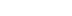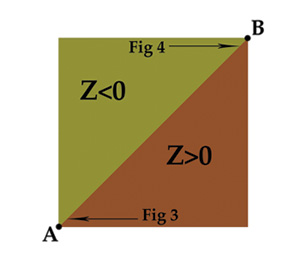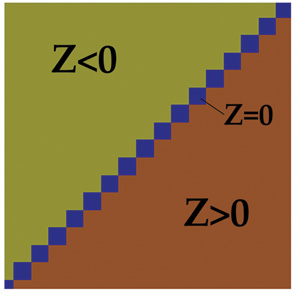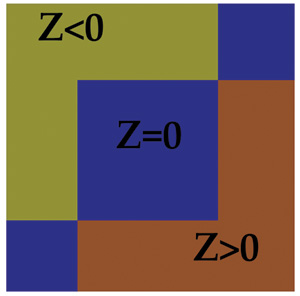/
article

# Visualizing Floats

Despite their ubiquity, few programmers really take the time to study the underlying mechanics of floating point numbers, their limitations, and problems they can bring to games -- programmer West dives in.

Floating point numbers permeate almost every area of game programming. They are used to represent everything from position, velocity, and acceleration, to fuzzy AI variables, texture coordinates, and colors. Yet, despite their ubiquitous role, few programmers really take the time to study the underlying mechanics of floating point numbers, their inherent limitations, and the specific problems these can bring to games.

This article explores some of the problems with floats, illustrating certain examples in the hope that programmers will be somewhat less surprised when these problems crop up mid-project. With any luck, you will be better equipped to visualize and deal with these and other related problems.

### What Is A Float?

The term "floating point number" can be used to describe many different kinds of number representation. But for game programmers, there are really only two that we need to be concerned with: single and double precision floating point numbers.

By far the most common is the single precision 32-bit floating point number, commonly referred to by its C keyword "float." Due to the convenient size and the requirements of the hardware, this is the most popular format for storing and manipulating numbers on all modern gaming platforms (although some platforms use 24-bit floats in part of their hardware graphics pipeline, which can greatly magnify the problems discussed below).

A float consists of 32 bits: a sign bit, an 8-bit exponent (e), and a 23-bit significand (s). For precise details, see References.

To visualize the problems with floats, it's useful to visualize the differences between floats and integers. Consider how the 32-bit integers represent space. There are 2^32 integers; each one can be thought of as representing a region between two points on a line. If each integer represents 1 millimeter, then you can represent any distance using integers from 1mm to 2^32mm. That's any distance up to about 4,295km, about 2,669 miles, with a resolution of 1mm.

Now picture how one might represent 2D space with integers. If you again consider a resolution of 1mm, you can represent any position in a 4,295x4,295 kilometer square area to a resolution of 1mm. Imagine zooming in closely and seeing the actual grid of integers.

Now take it one more step and use the same setup to represent 3D space. This time each individual position can be thought of as the space within tiny 1mm cubes, so full 3D space is made up of a grid of these identically sized cubes.Figure 1: A 3D shape is represented in floats as a series of cubic volumes.

You can't represent anything smaller than 1mm, and objects that are only a few millimeters in size will have a blocky appearance. Figure 1 represents the general idea.

The important thing to remember about these integer-defined cubes is that they are all the same size. In 3D space, the cubes of space near the origin are the same as the cubes of space a mile away from the origin.

### Floats vs. Integers

Let's compare the 3D integer arrangement to floats. First off, note that both integers and floats (in practice) are stored as 32-bit words. Since there are only 2^32 possible bit patterns, that means the number of possible floats is the same as the number of possible integers. Yet floating point numbers can represent numbers in a range from 0 to 2^128. [Note: There are actually a few less floats, as some float bit patterns are "not a number" (NaN), but we'll ignore that for simplicity's sake. For the purpose of this article, I will also simplify the treatment of signed quantities.]

How this larger range of numbers works is fairly obvious if you study the representation of a float. Still, it's useful to look into this to gain an understanding of what's going on.

The key thing to note is that there is the same number of floating point numbers between each power of two. So from 1 to 2 there are 8,388,608 (or 2^23) possible different floating point numbers, and from 2 to 4 there is the same total number. There's also the same number of possible floats between 32,768 and 65,536, or 0.03125 and 0.0625.

Here's another way of thinking about it: If you represent a position with a floating point number, then there are more possible points between the origin and a point 1mm away than there are possible points between the origin and a point on the other side of the planet. This means the precision of your floating point representation of a position depends on where you're standing and what units you're using.

If, again, a floating point value of 1.0 represents 1mm, then when you stand near the origin (meaning your represented position is close to 0,0,0) your position can be represented to an accuracy of about 0.0000001mm, which is incredibly precise.

However, as you move away from the origin, your accuracy begins to decrease. At only 1 kilometer away from the origin (1,000,000mm), the accuracy drops to 0.125mm, which is still pretty good. But if you move even farther to a distance of 64km from the origin, the accuracy drops precipitously to 4mm, which means you can only represent a position with an accuracy of 4mm-a quarter of the resolution the integers could detect.

It gets worse. If you travel farther out to the edge of the space that could be represented with integers, at 4,295km (roughly the distance from Los Angeles to New York), you are at 2^32mm; yet, since we can only represent 2^23-bits of precision, our accuracy drops to 29mm, or 512mm-about half a meter.

So if you used 32-bit floats to represent positions in a game that spanned the continental U.S., then on one coast your positions can only be represented with an accuracy of half a meter (1.5 feet), and clearly, that is unacceptable.

### Some Solutions

Use relative coordinates. The origin in your universe is in a fixed position, but you can perform all your calculations in a space relative to an origin closer to the action, such as the camera viewpoint. Positions themselves can be stored as floats relative to some other local origin, whose position relative to the universe origin is defined in a more accurate manner.

Use fixed points. If it's important to your game that everything look and act the same whether near the origin or far away from it, then you can use fixed-point numbers to store your positions.

Essentially, it's like using integers but with a sufficiently small unit, so for example 1 could represent 0.1mm, or whatever works for your situation. This can be extended to use 64-bit fixed points for even greater range and accuracy.

Use doubles. For defining points that are a long way from the origin, you can use double precision floating point numbers. You can either define all positions as doubles and then convert to a local space for manipulation as floats, or define a remote region's position using doubles and use relative positions within that space using floats.

### Boundary Conditions

We often think of polygons and their edges as pure mathematical planes and lines, which is useful when formulating algorithms to solve certain problems. Consider a simple 2D problem: deciding which side of a line a point is on.

This kind of test is often used when determining if a point is inside a triangle or other similar tasks. So, we specify it mathematically: Given a line formed by two points A and B, and a third point P, we calculate the Z component of the cross product of AP and AB, Z, such that Z=((P-A)x(B-A)).z.

If Z is negative, then C is on the left, and if Z is positive, it's on the right of the line. This relationship is purely mathematical.

To see if a point is inside a 2D triangle, a simple method is to traverse the points of the triangle in a clockwise order and use the same test to see if the point is to the right of each of the three edges of the triangle.

This test can also be used for 3D line-triangle collision detection by first transforming the triangle's points into the space of the collision line (using the transform that would make the line parallel to the z-axis, reducing the problem to two dimensions).Figure 2: The line from A=(0,0) to B=(5000,5000) separates all points P in this region into two triangles based on the sign of z of the cross product APxAB.

If we have two triangles that share an edge (as most triangles do in video games), and we apply the above tests to them, we should be able to accurately determine which triangle a line lays on. Figure 2 shows two triangles and the results of the test (Z<0) on the line AB that defines the edge they share. What a nice, clean, mathematical split.

Of course, the obvious problem with this test is for points that lay on the line between the polygons, where Z=0. In our pure mathematical world, a line is an infinitely thin region between the polygons. But in the practical world of floating points, the reality is rather different.Figure 3: In the region x and y from 800.0 to 800.001 there are a number of indeterminate regions between the triangles.

If you zoom in on the line, down to the level of the individual float regions I described earlier, you will see the line defined by Z=0 is composed of a series of regions (see Figure 3). What's more, if you zoom in on the same line, but go farther from the origin, you see that the size of these regions increases (as Figure 4 illustrates).Figure 4: At a different position on the same edge 9x and y from 4,800.0 to 4,800.001) the indeterminate regions are much larger.

The result of this could go two ways depending on how you implement your logic. If you started out saying, "Z>0 implies the point if to the left of the line," then all the floating point regions that are on the line (Z=0), will show up as little holes-regions where the collision fails. The quick solution here is to change the test to Z greater than or equal to 0. This eliminates the problem of holes but creates a new problem-the regions on the line (Z=0) are now shared by both triangles.

Problems can arise if the collision routine returns a list of all the triangles it detects a collision with. The logic might not be set up to deal with having contact with two different surfaces in the same logic frame, leading to problems such as sound effects being stuck on or events failing to trigger.

More commonly though, a line-environment collision test will return the closest collision point. Since both polygons will return the same point (which, as we see in the figures, is actually an overlapping region), then the polygon detected will be determined by the order in which the polygons are tested.

Historically, the polygons are tested in the same order. However, with the increasing prevalence of multi-core architectures, it's increasingly common for programmers to implement some kind of data-level parallelism, where the order in which the individual polygons are tested is not guaranteed and varies based on the way additional tasks use the cores and by the state of the memory cache, which varies from frame to frame.

The result can be that the same collision test performed on the same data might return either of two polygons in a seemingly random manner. Most likely, it will return one polygon 99.99 percent of the time, with the other polygon cropping up extremely rarely.

You might even find a Heisenbug, which can be incredibly difficult to track down, since a) it surfaces very rarely, b) the conditions can be impossible to replicate, and c) introducing test code can "fix" the problem.

There are a number of solutions. For one, you can change your multi-core data sharing algorithm so that polygons which might share an edge are always submitted in the same batch-but it would still leave you with the potential problem of two polygons being returned with the same collision point.

You could also try to guarantee that the regions on the line Z=0 always belong to one polygon of the other, which you could do by flagging the edges of a polygon so one side uses Z<0 and the other effectively uses Z greater than or equal to 0.

### Pinning Down Drifters

Floats are a very useful way of representing numbers, but keep in mind that they do not perfectly represent the mathematical world that you use when creating algorithms. Floating point coordinates represent regions in space rather than points.

Those regions get a lot bigger as you move farther from the origin and eventually create noticeable artifacts such as jittering and visible seams. This is an important consideration if you are attempting to scale an existing engine to one that supports a much large world.

Floating point inaccuracies can lead to indeterminate boundary regions of variable size, and these need to be dealt with explicitly to avoid Heisenbugs.

### References

Ericson, Christer. "Numerical Robustness" in Real-Time Collision Detection. San Francisco: Morgan Kaufmann, 2004.

Freese, Peter. "Solving Accuracy Problems in Large World Coordinates" in Game Programming Gems 4, Hingham, Mass.: Charles River Media, 2004.

[EDITOR'S NOTE: This article was independently published by Gamasutra's editors, since it was deemed of value to the community. Its publishing has been made possible by Intel, as a platform and vendor-agnostic part of Intel's Visual Computing microsite.]

### Latest Jobs

#### Treyarch

Playa Vista, California
6.20.22
Audio Engineer

#### Digital Extremes

6.20.22
Communications Director

6.20.22
Senior Producer

#### Build a Rocket Boy Games

Edinburgh, Scotland
6.20.22
More Jobs